Vibroengineering PROCEDIA

Published: 28 November 2019

# Free vibration of circular annular plate with different boundary conditions

Yash Jaiman1
Baij Singh2
1Bennett University, Greater Noida, Uttar Pradesh, India
2Indian Institute of Technology (ISM), Dhanbad, India
Corresponding Author:
Yash Jaiman
Views 668

#### Abstract

This paper deals with the numerical simulation of free vibration analysis of a thin circular annular plate for various boundary conditions at the outer edge and inner edge. Classical plate theory is used to derive the governing differential equation for the transverse deflection of the thin isotropic plate. The finite element method is used to evaluate the first six natural frequencies and mode shapes of the thin uniform circular annular plate with radius ratios $\left({r}_{1}/{r}_{2}\right)$ for different boundary conditions. These natural frequencies results are compared with those available in the literature. The results are verified with classical plate theory with our Abaqus results and checked with the previous research literature on the topic.

## 1. Introduction

Plates are widely used as a structural element and have vast practical applications in many engineering fields such as aerospace, mechanical, civil, nuclear, electronic, automotive, marine and heavy machinery, etc. Various researchers have analyzed the free vibration behavior of circular annular plates of different shapes, sizes, thickness for different boundary conditions. Leissa  used the Ritz method to estimate the natural frequencies of the isotropic plate for different boundary conditions. Kim and Dickinson  used the Rayleigh-Ritz approximation method for free vibration of a thin plate to extract natural frequencies. Rajalingham et al.  used a Rayleigh-Ritz method to analyze the plate characteristics parameter as shape functions and continued his work to formulate a variational reduction expression to analyze frequencies and mode shapes. Liew et al.  used the polynomials-Ritz method for the vibration of circular plates by using three-dimensional elasticity solutions. Zhou et al.  used the Chebyshev-Ritz method for three-dimensional vibration and mode shapes of the circular plate. Lim et al.  used the state-space method to analyze transverse vibration and mode of a thick circular plate. Zhou et al.  used the Hamiltonian principle to solve governing equations for free vibration analysis by using the variational principle of mixed energy method. Kumar et al.  use a dynamic stiffness method to extract the natural frequency and mode shapes of a thin plate. Piyush et al.  used the Rayleigh-Ritz method to compute the natural frequencies of the thin plate.

## 2. Basic formulation

Consider a homogeneous, isotropic circular annular plate in cylindrical coordinates $\left(r,\theta ,z\right)$ with uniform thickness $h$ as shown in Fig. 1.

Classical plate theory is used to derive the governing differential equation for transverse vibration in the polar coordinate system is defined as:

1

where Laplacian operator: ${\nabla }^{2}=\frac{{\partial }^{2}}{{\partial r}^{2}}+\frac{1}{r}\frac{\partial }{\partial r}+\frac{1}{{r}^{2}}\frac{{\partial }^{2}}{{\partial \theta }^{2}}$, $\rho$ is the mass density, $D=E{h}^{3}/\left[12\left(1-{\nu }^{2}\right)\right]$ is the flexural rigidity and $\nu$ is Poisson’s ratio.

Transverse deflection of natural vibrations for thin circular plate is assumed to be:

2
$W\left(r,\theta ,t\right)=w\left(r,\theta \right){e}^{i\omega t},$

where $\omega$ is the natural frequency and $w\left(r,\theta \right)$ is natural mode.

Substituting Eqs. (2) in (1), we get:

3

where:

4
${\gamma }^{4}=\frac{\rho h{\omega }^{2}}{D}.$

The general finite element equation for the transverse deflection of thin plate is given by:

5
$\left[M\right]\left\{\stackrel{¨}{q}\right\}+\left[K\right]\left\{q\right\}=0,$

where $\left[M\right]$ is the mass matrix, $\left[K\right]$ is stiffness matrix, $\left\{\stackrel{¨}{q}\right\}$ is the nodal acceleration vector, and $\left\{q\right\}$ is nodal displacement vector.

The non-dimension natural frequency parameter $\varpi$ are calculated as:

6
$\varpi =2\pi \omega \left[\sqrt{\rho h/D}\right]{r}_{1}^{2},$

where $\omega$ is the natural frequency in Hz.

Fig. 1Schematic diagram and coordinate system of annular circular plate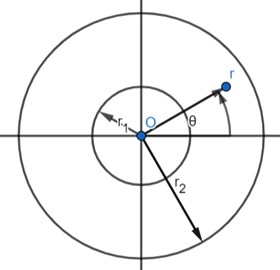## 3. Results and discussions

In this section, the first six non-dimensional natural frequencies and mode shapes of the circular annular plate are estimated by using the finite element method. Here, we calculated different eigenvalues for different boundary conditions with different radii ratio $\left({r}_{1}/{r}_{2}\right)$. Different combinations of boundary conditions are applied to compute the natural frequencies and mode shapes of the circular annular plate. 2820 elements and 5922 nodes are used to estimate the natural frequencies and mode shape function of thin circular annular plates after convergence study. The present natural frequencies results are compared with those available in the literature.

Tables 1-4 shows that the first six non-dimensional natural frequencies values for the circular annular plate. These present results are nearly the same as Leissa  and Zhou  under different boundary conditions.

Fig. 2Natural modes of a clamped annular plate with a free inner boundary, r1/r2 = 0.4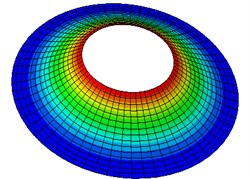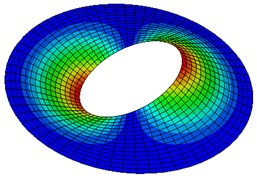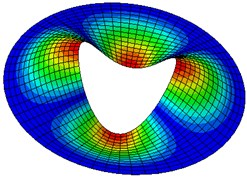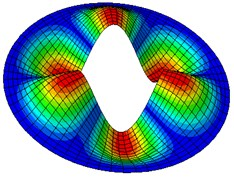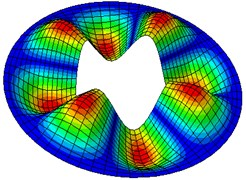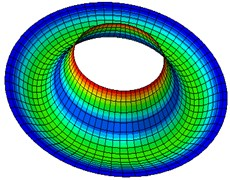Table 5-7 presents the effect of the radii ratio on the non-dimensional frequency parameter of thin plates. It is observed from these tables that as the radii ratio increases non-dimensional frequency parameter increases.

Table 1Comparison of the non-dimensional natural frequency parameter with Leissa  and Zhou  for clamped outer and free inner boundary (ν=1/3, r1/r2 =0.4)

 Results Mode number 1 2 3 4 5 6 Leissa  13.54 19.80 31.34 – – – Zhou  13.500 19.389 31.338 46.855 65.984 66.924 Present 12.871 18.497 29.901 44.70 62.982 63.935

Table 2Comparison of the non-dimensional natural frequency parameter with Leissa  and Zhou  for free outer and clamped inner boundary (ν=1/3, r1/r2 =0.4)

 Results Mode number 1 2 3 4 5 6 Leissa  9.096 10.37 – – – – Zhou  9.0719 9.1294 10.366 14.726 22.530 33.455 Present 9.0257 9.0868 10.3267 14.6805 22.5682 33.4769

Table 3Comparison of the non-dimensional natural frequency parameter with Leissa  and Zhou  for free outer and free inner boundary (ν=1/3, r1/r2 =0.4)

 Results Mode number 1 2 3 4 5 6 Leissa  – 4.567 – – – – Zhou  4.5325 8.5510 11.765 17.043 21.262 31.356 Present 4.5197 8.5070 11.7368 16.9765 21.233 31.2565

Table 4Comparison of the non-dimensional natural frequency parameter with Leissa  and Zhou  for clamped outer and clamped inner boundary (ν=1/3, r1/r2 =0.4)

 Results Mode number 1 2 3 4 5 6 Leissa  62.33 62.92 66.406 – – – Zhou  61.872 62.966 66.672 73.630 84.594 99.904 Present 62.0056 63.1121 66.7350 73.6148 84.5044 99.7988

Table 5Non-dimensional frequency parameter ωr22ρh/D  for the annular circular plate with clamped outer and free inner edge (ν=1/3)

 $n$ ${r}_{1}/{r}_{2}$ 0.2 0.4 0.6 0.8 0 10.2922 12.871 25.3966 92.3617 1 20.4306 18.497 28.3519 94.2334 2 33.6865 29.001 36.2745 98.3097 3 50.4963 44.70 47.9049 105.6264 4 69.6342 62.982 62.8458 115.7173 5 91.0597 83.751 79.4797 128.4994

Table 6Non-dimensional frequency parameter ωr22ρh/D  for the annular circular plate with free outer and clamped inner edge (ν=1/3)

 $n$ ${r}_{1}/{r}_{2}$ 0.2 0.4 0.6 0.8 0 4.795 9.0257 20.5213 84.5418 1 5.190 9.0868 20.8923 85.1491 2 6.322 10.3267 22.3356 87.0250 3 12.367 14.6805 25.6329 90.3110 4 21.502 22.5682 31.5306 95.2026 5 32.438 33.4769 40.3467 101.941

Table 7Non-dimensional frequency parameter ωr22ρh/D  for the annular circular plate with clamped outer and clamped inner edge (ν=1/3)

 $n$ ${r}_{1}/{r}_{2}$ 0.2 0.4 0.6 0.8 0 4.795 9.0257 141.4272 593.2696 1 5.190 9.0868 144.8754 593.8935 2 6.322 10.3267 149.467 595.8484 3 12.367 14.6805 156.3015 599.0929 4 21.502 22.5682 165.7894 603.7515 5 32.438 33.4769 178.2596 609.866

## 4. Conclusions

In this paper, numerical analysis for free vibration analysis of a thin annular solid plate is carried out using the finite element method for different boundary conditions at the inner and outer radius. It is found that those natural frequency results are quite close to those reported in previous works of literature. The novelty of this paper is the effect of the radii ratio on natural frequency is discussed and found that with increasing radii ratio, natural frequency increases and another novelty is by using shell element modeling as per Abaqus convention the dimensionless frequency parameter as found in the literature are completely validated.

#### Cited by

Study of modes and deflection for ring shaped plate with varying thickness
Analysis of the dynamical behaviour of spinning annular disks with various boundary conditions